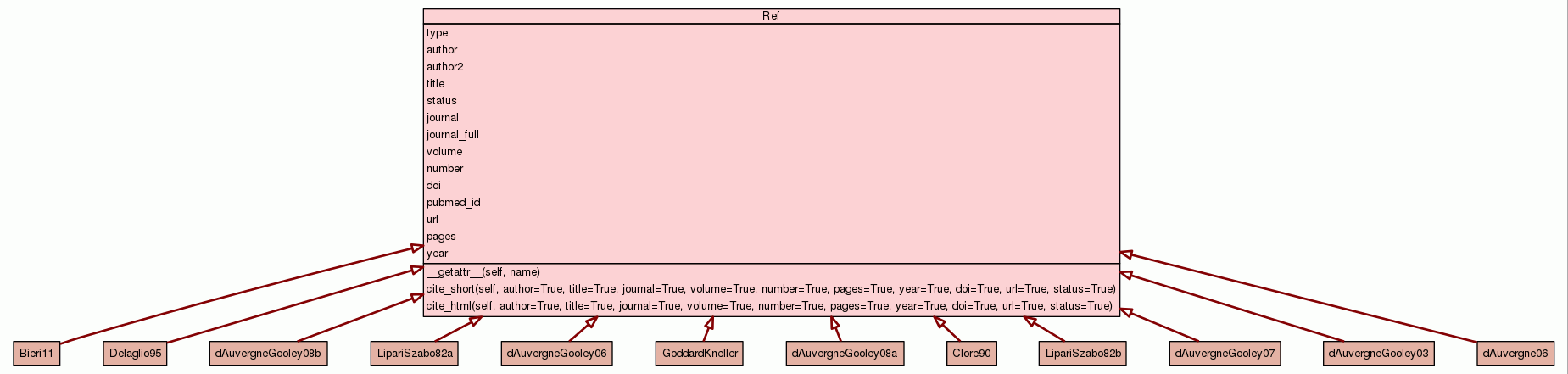[frames] | no frames]

# Class Ref

source codeReference base class.

 Instance Methods
anything
 __getattr__(self, name) Generate some variables on the fly. source code
str
 cite_short(self, author=True, title=True, journal=True, volume=True, number=True, pages=True, year=True, doi=True, url=True, status=True) Compile a short citation. source code
str
 cite_html(self, author=True, title=True, journal=True, volume=True, number=True, pages=True, year=True, doi=True, url=True, status=True) Compile a citation for HTML display. source code
 Class Variables
type = `None`
hash(x)
author = `None`
hash(x)
author2 = `None`
hash(x)
title = `None`
hash(x)
status = `None`
hash(x)
journal = `None`
hash(x)
journal_full = `None`
hash(x)
volume = `None`
hash(x)
number = `None`
hash(x)
doi = `None`
hash(x)
pubmed_id = `None`
hash(x)
url = `None`
hash(x)
pages = `None`
hash(x)
year = `None`
hash(x)
 Method Details

### __getattr__(self, name)(Qualification operator)

source code

Generate some variables on the fly.

Parameters:
• `name` (str) - The name of the object.
Returns: anything
The generated object.
Raises:
• `AttributeError` - If the object cannot be created.

### cite_short(self, author=True, title=True, journal=True, volume=True, number=True, pages=True, year=True, doi=True, url=True, status=True)

source code

Compile a short citation.

The returned text will have the form of:

• d'Auvergne, E.J. and Gooley, P.R. (2008). Optimisation of NMR dynamic models I. Minimisation algorithms and their performance within the model-free and Brownian rotational diffusion spaces. J. Biomol. NMR, 40(2), 107-119.
Parameters:
• `author` (bool) - The author flag.
• `title` (bool) - The title flag.
• `journal` (bool) - The journal flag.
• `volume` (bool) - The volume flag.
• `number` (bool) - The number flag.
• `pages` (bool) - The pages flag.
• `year` (bool) - The year flag.
• `doi` (bool) - The doi flag.
• `url` (bool) - The url flag.
• `status` (bool) - The status flag. This will only be shown if not 'published'.
Returns: str
The full citation.

### cite_html(self, author=True, title=True, journal=True, volume=True, number=True, pages=True, year=True, doi=True, url=True, status=True)

source code

Compile a citation for HTML display.

Parameters:
• `author` (bool) - The author flag.
• `title` (bool) - The title flag.
• `journal` (bool) - The journal flag.
• `volume` (bool) - The volume flag.
• `number` (bool) - The number flag.
• `pages` (bool) - The pages flag.
• `year` (bool) - The year flag.
• `doi` (bool) - The doi flag.
• `url` (bool) - The url flag.
• `status` (bool) - The status flag. This will only be shown if not 'published'.
Returns: str
The full citation.

 Generated by Epydoc 3.0.1 on Fri Jun 14 11:29:30 2019 http://epydoc.sourceforge.net